1. Home
2. Yarn Engineering
3. Textile Physics-1
4. Flexural Properties
5. Flexural Properties of Textile Materials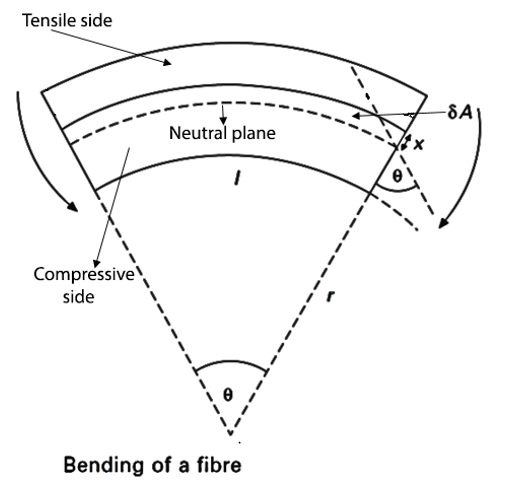# Flexural Properties of Textile Materials

3.51K
0#### Md Sohanur Rahman Sobuj

Co-founder , Admin & Author at Textile Study Center
Student of Bangladesh University of Textiles (BUTEX) Department: Apparel Engineering#### Latest posts by Md Sohanur Rahman Sobuj (see all)

Flexural Properties of Textile Materials

The behavior which shows by textile material during bending is called flexural (bending) property. The importance of Flexural properties is required when we wear cloth.

Significance of Bending and Twisting:

The bending and twisting of fibers influence the behavior of bulked yarn and the drape and handle of fabrics.

Twisting and bending both play a part in the arrangement of fibers in a yarn.

Bending strength and shear strength may be important in wear.

Recovery from bending is a factor of creasing.

Flexural Rigidity:

Flexural rigidity is a measure of stiffness associated with handle. It is defined as the couple required to bend the fiber to unit curvature. Bending rigidity is inversely proportional to the square of the radius. So, finer fibers are easier to bend.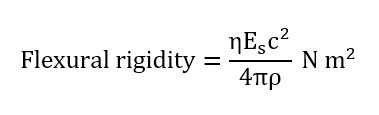Where, Es specific modulus (N/kg m)

h  fiber shape factor (=1 for a circular fiber)

=   fiber linear density (kg/m3)

=   fiber density (kg/m3)

In Practical Units, the Equation Becomes:Specific Flexural Rigidity :

Specific flexural rigidity is defined as the flexural rigidity of a fiber in linear density.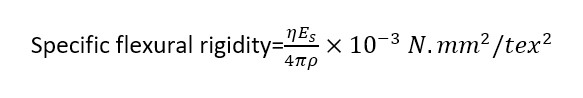Flexural/Bending Rigidity for a Small Curvature:

Suppose a specimen of length l, bent through an angle θ to a radius of curvature r as shown in fig. Outer layers of the fiber are stretched and the inner layers are compressed. While bending bent, the length of the tensile side of the fiber increases, but the length of the compressive side decreases. However, the neural plane between the tensile and compressive sides remains unchanged in length. As a result of the extension and compression, stresses will be set up that give an internal couple to balance the applied couple.

Consider an element of area of cross-section δA, at a perpendicular distance x from the neutral plane: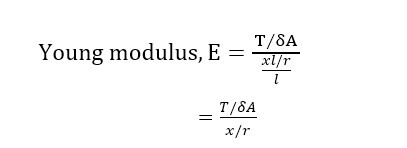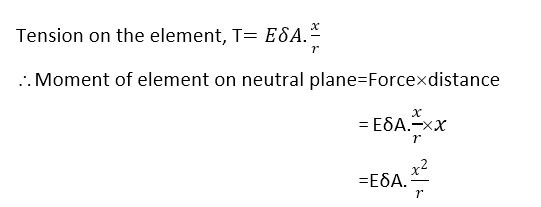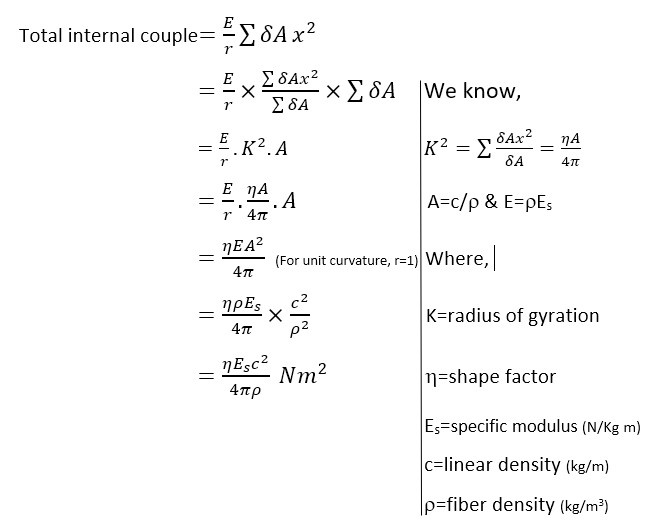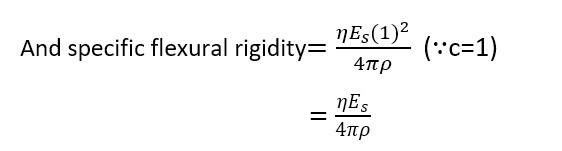So, the flexibility of a fiber depends on its shape, its tensile modulus, its density (1.1-1.6g/cm3) and, most of all, its thickness.

Bending Recovery :

The recovery from a given curvature is called bending recovery. 15 denier (15D) nylon fiber shows 100% recovery from small curvature and 20% recovery from large curvature.

Unit = N.m2/ Tex.

Shape Factor (h) :

Shape factor is a number that indicates the shape of a fiber. Shape is expressed by “η”.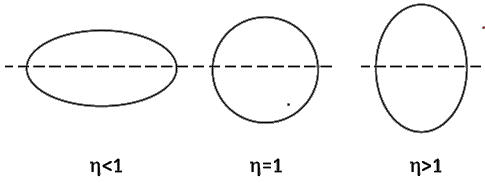⦿ If, η = 1, it indicates the shape of fiber is circular.

⦿ If, η > 1, it indicates the shape of fiber is increased.

⦿ If, η < 1, it indicates the shape of fiber is decreased.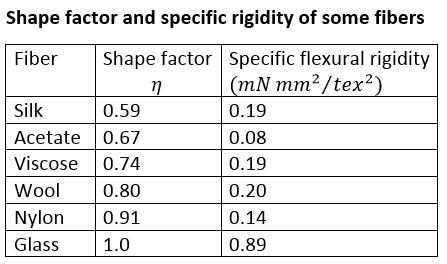Mathematical Problems with Solutions :

Required laws: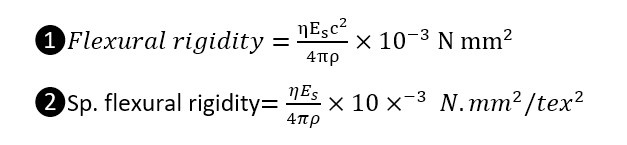Where, Es =  Specific modulus (N/tex)

c   =  linear density (gm/cc)

r   =  fiber density (gm/cc)

h  =  Shape factor

Problem 01: Find out the flexural rigidity of a circular fiber, where the linear density, specific modulus and density of that fiber are 3.5 tex, 5 N/tex and 1.1 gm/cc respectively.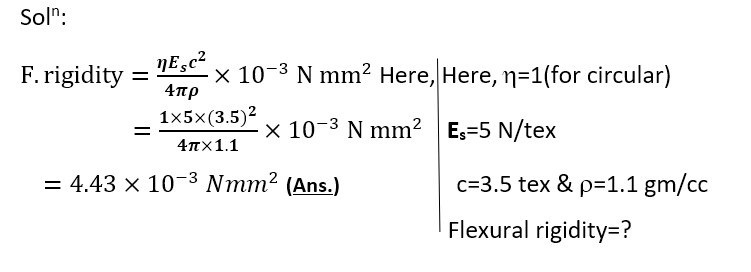Problem 02: Find out the flexural rigidity of viscose fiber where specific modulus= 0.2´10-3 n/tex, Linear density 0.2 tex, Density=1.12 gm/cc, shape factor=0.74.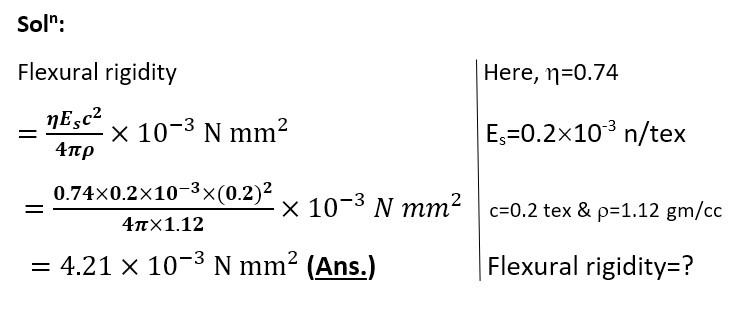(3513)#### Md Sohanur Rahman Sobuj

Co-founder , Admin & Author at Textile Study Center
Student of Bangladesh University of Textiles (BUTEX) Department: Apparel Engineering#### Latest posts by Md Sohanur Rahman Sobuj (see all)Close
Share via